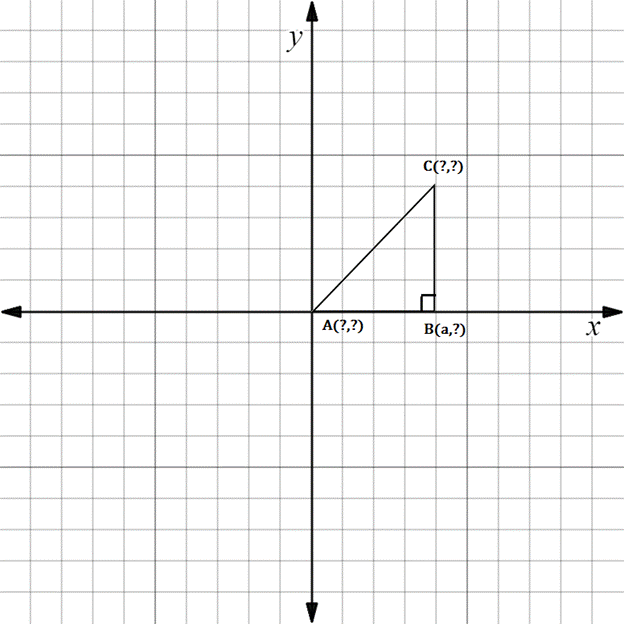Chapter 10.3, Problem 11EElementary Geometry For College St...

7th Edition
Alexander + 2 others
ISBN: 9781337614085

Solutions

Chapter
SectionElementary Geometry For College St...

7th Edition
Alexander + 2 others
ISBN: 9781337614085
Textbook Problem

In Exercises 11 to 16, supply the missing coordinates for the vertices, using as few variables as possible.A B C is a right triangle

To determine

To find:

The missing coordinates for the vertices.

Explanation

The given figure is shown below.

From the figure, the following observations are made.

Since, the vertex A is at the origin.

The coordinates of A0, 0.

Now, consider the vertex B.

Here, x-coordinate is given as a.

In the B vertex, observe where it lies on y-axis.

Since, it is at 0, the vertex B has the coordinates a, 0.

The distance from A to B in the x-axis is a.

Hence, the distance from origin to C in the x-axis is also a

Still sussing out bartleby?

Check out a sample textbook solution.

See a sample solution

The Solution to Your Study Problems

Bartleby provides explanations to thousands of textbook problems written by our experts, many with advanced degrees!

Get Started

Evaluate each expression: 32+12323

Elementary Technical Mathematics

In Exercises 3540, rationalize the numerator of each expression. 38. x1x

Applied Calculus for the Managerial, Life, and Social Sciences: A Brief Approach

Evaluate the definite integral. 12xx1dx

Single Variable Calculus: Early Transcendentals, Volume I

Finding Intercepts In Exercises 19-28, find any intercepts. y2=x34x

Calculus: Early Transcendental Functions (MindTap Course List)